UPSC  >  Test: Letter Series Type 2

# Test: Letter Series Type 2

Test Description

## 30 Questions MCQ Test Logical Reasoning (LR) and Data Interpretation (DI) | Test: Letter Series Type 2

Test: Letter Series Type 2 for UPSC 2022 is part of Logical Reasoning (LR) and Data Interpretation (DI) preparation. The Test: Letter Series Type 2 questions and answers have been prepared according to the UPSC exam syllabus.The Test: Letter Series Type 2 MCQs are made for UPSC 2022 Exam. Find important definitions, questions, notes, meanings, examples, exercises, MCQs and online tests for Test: Letter Series Type 2 below.
Solutions of Test: Letter Series Type 2 questions in English are available as part of our Logical Reasoning (LR) and Data Interpretation (DI) for UPSC & Test: Letter Series Type 2 solutions in Hindi for Logical Reasoning (LR) and Data Interpretation (DI) course. Download more important topics, notes, lectures and mock test series for UPSC Exam by signing up for free. Attempt Test: Letter Series Type 2 | 30 questions in 25 minutes | Mock test for UPSC preparation | Free important questions MCQ to study Logical Reasoning (LR) and Data Interpretation (DI) for UPSC Exam | Download free PDF with solutions
 1 Crore+ students have signed up on EduRev. Have you?
Test: Letter Series Type 2 - Question 1

### Directions to Solve In each of the following questions, various terms of an alphabet series are given with one or more terms missing as shown by (?). Choose the missing terms out of the given alternatives. Question - WFB, TGD, QHG, ?

Detailed Solution for Test: Letter Series Type 2 - Question 1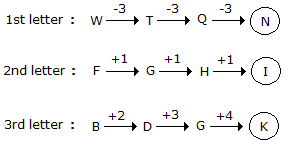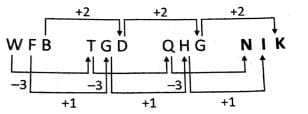Test: Letter Series Type 2 - Question 2

### Directions to Solve In each of the following questions, various terms of an alphabet series are given with one or more terms missing as shown by (?). Choose the missing terms out of the given alternatives. Question - AZ, GT, MN, ?, YB

Detailed Solution for Test: Letter Series Type 2 - Question 2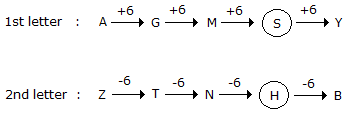Test: Letter Series Type 2 - Question 3

### Directions to Solve In each of the following questions, various terms of an alphabet series are given with one or more terms missing as shown by (?). Choose the missing terms out of the given alternatives. Question - H, I, K, N, ?

Detailed Solution for Test: Letter Series Type 2 - Question 3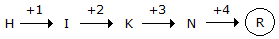Test: Letter Series Type 2 - Question 4

Directions to Solve

In each of the following questions, various terms of an alphabet series are given with one or more terms missing as shown by (?). Choose the missing terms out of the given alternatives.

Question -

Z, S, W, O, T, K, Q, G, ?, ?

Detailed Solution for Test: Letter Series Type 2 - Question 4

The given sequence is a combination of two series:

I. Z, W, T, Q, ? and II. S, O, k, G, ?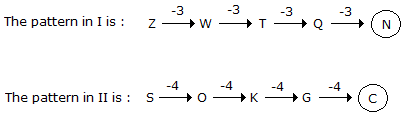Test: Letter Series Type 2 - Question 5

Directions to Solve

In each of the following questions, various terms of an alphabet series are given with one or more terms missing as shown by (?). Choose the missing terms out of the given alternatives.

Question -

b e d f ? h j ? l

Detailed Solution for Test: Letter Series Type 2 - Question 5

The series may be divided into groups as shown:

b e d / f ? h / j ? l

Clearly in the first group, the second and third letters are respectively three and two steps ahead of the first letter.

A similar pattern would follow in the second and third groups.

Test: Letter Series Type 2 - Question 6

Directions to Solve

In each of the following questions, various terms of an alphabet series are given with one or more terms missing as shown by (?). Choose the missing terms out of the given alternatives.

Question -

A, G, L, P, S, ?

Detailed Solution for Test: Letter Series Type 2 - Question 6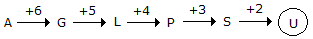Test: Letter Series Type 2 - Question 7

Directions to Solve

In each of the following questions, various terms of an alphabet series are given with one or more terms missing as shown by (?). Choose the missing terms out of the given alternatives.

Question -

ajs, gpy, ?, sbk, yhq

Detailed Solution for Test: Letter Series Type 2 - Question 7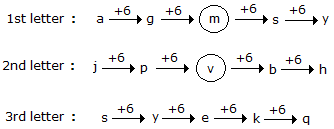Test: Letter Series Type 2 - Question 8

Directions to Solve

In each of the following questions, various terms of an alphabet series are given with one or more terms missing as shown by (?). Choose the missing terms out of the given alternatives.

Question -

Y, B, T, G, O, ?

Detailed Solution for Test: Letter Series Type 2 - Question 8

The given sequence is a combination of two series :

I. Y,T, O and II. B, G, ?

I consists of 2nd, 7th and 12th letters from the end of the English alphabet, while

II consists of 2nd, 7th and 12th letters from the beginning of the English alphabet.

So, the missing letter in II is the 12th letter from the beginning of the English alphabet, which is L.

Test: Letter Series Type 2 - Question 9

Directions to Solve

In each of the following questions, various terms of an alphabet series are given with one or more terms missing as shown by (?). Choose the missing terms out of the given alternatives.

Question -

AB, DEF, HIJK, ?, STUVWX

Detailed Solution for Test: Letter Series Type 2 - Question 9

The number of letters in the terms of the given series increases by one at each step.

The first letter of each term is two steps ahead of the last letter of the preceding term.

However, each term consists of consecutive letters in order.

Test: Letter Series Type 2 - Question 10

Directions to Solve

In each of the following questions, various terms of an alphabet series are given with one or more terms missing as shown by (?). Choose the missing terms out of the given alternatives.

Question -

C, Z, F, X, I, V, L, T, O, ?, ?

Detailed Solution for Test: Letter Series Type 2 - Question 10

The given sequence is a combination of two series:

I. C, F, I, L, O, ? and II. Z, X, V, T, ?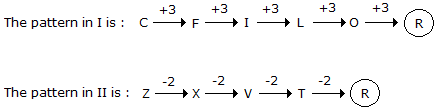Test: Letter Series Type 2 - Question 11

Directions to Solve

In each of the following questions, various terms of an alphabet series are given with one or more terms missing as shown by (?). Choose the missing terms out of the given alternatives.

Question -

A, B, B, D, C, F, D; H, E, ?, ?

Detailed Solution for Test: Letter Series Type 2 - Question 11

The given sequence is a combination of two series :

I. 1st, 3rd, 5th, 7th, 9th, 11th terms i.e. A, B, C, D, E, ? II. 2nd, 4th, 6th, 8th, 10th terms i.e. B, D, F, H, ?

Clearly, I consists of consecutive letters while II consists of alternate letters. So, the missing letter in I is F, while that in II is J. So, the missing terms i.e. 10th and 11th terms are J and F respectively.

Test: Letter Series Type 2 - Question 12

Directions to Solve

In each of the following questions, various terms of an alphabet series are given with one or more terms missing as shown by (?). Choose the missing terms out of the given alternatives.

Question -

Z, X, S, I, R, R, ?, ?

Detailed Solution for Test: Letter Series Type 2 - Question 12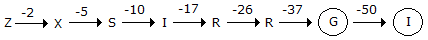Note that the numbers representing the difference between the consecutive terms of the series again from a series - 2, 5, 10, 17, 26, 37, 50 - in which the pattern is +3, +5, +7, +9, +11, +13.

Test: Letter Series Type 2 - Question 13

Directions to Solve

In each of the following questions, various terms of an alphabet series are given with one or more terms missing as shown by (?). Choose the missing terms out of the given alternatives.

Question -

T, R, P, N, L, ?, ?

Detailed Solution for Test: Letter Series Type 2 - Question 13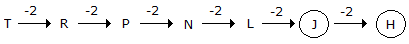Test: Letter Series Type 2 - Question 14

Directions to Solve

In each of the following questions, various terms of an alphabet series are given with one or more terms missing as shown by (?). Choose the missing terms out of the given alternatives.

Question -

AYBZC, DWEXF, GUHVI, JSKTL, ?

Detailed Solution for Test: Letter Series Type 2 - Question 14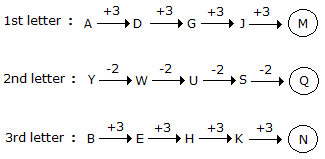Test: Letter Series Type 2 - Question 15

Directions to Solve

In each of the following questions, various terms of an alphabet series are given with one or more terms missing as shown by (?). Choose the missing terms out of the given alternatives.

Question -

PMT, OOS, NQR, MSQ, ?

Detailed Solution for Test: Letter Series Type 2 - Question 15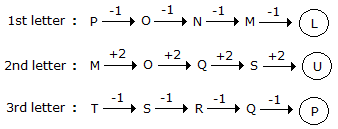Test: Letter Series Type 2 - Question 16

Directions to Solve

In each of the following questions, various terms of an alphabet series are given with one or more terms missing as shown by (?). Choose the missing terms out of the given alternatives.

Question -

A, B, N, C, D, O, E, F, P, ?, ?, ?

Detailed Solution for Test: Letter Series Type 2 - Question 16

The given series may be divided into 2 groups :

I. A, B, C, D, E, F, ?, ? and II. N, O, P, ?

Clearly, the given series consists of two terms of I followed by one term of II.

The missing terms in I are G and H while the missing term in II is Q.

Test: Letter Series Type 2 - Question 17

Directions to Solve

In each of the following questions, various terms of an alphabet series are given with one or more terms missing as shown by (?). Choose the missing terms out of the given alternatives.

Question -

GH, JL, NQ, SW, YD, ?

Detailed Solution for Test: Letter Series Type 2 - Question 17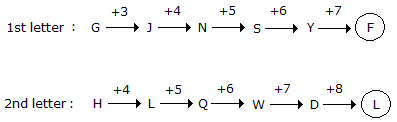Test: Letter Series Type 2 - Question 18

Directions to Solve

In each of the following questions, various terms of an alphabet series are given with one or more terms missing as shown by (?). Choose the missing terms out of the given alternatives.

Question -

R, U, X, A, D, ?

Detailed Solution for Test: Letter Series Type 2 - Question 18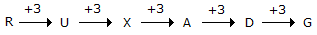Test: Letter Series Type 2 - Question 19

Directions to Solve

In each of the following questions, various terms of an alphabet series are given with one or more terms missing as shown by (?). Choose the missing terms out of the given alternatives.

Question -

AYD, BVF, DRH, ?, KGL

Detailed Solution for Test: Letter Series Type 2 - Question 19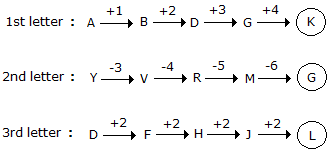Test: Letter Series Type 2 - Question 20

Directions to Solve

In each of the following questions, various terms of an alphabet series are given with one or more terms missing as shown by (?). Choose the missing terms out of the given alternatives.

Question -

AZ, CX, FU, ?

Detailed Solution for Test: Letter Series Type 2 - Question 20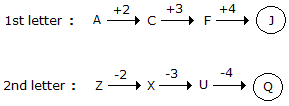Test: Letter Series Type 2 - Question 21

Directions to Solve

In each of the following questions, various terms of an alphabet series are given with one or more terms missing as shown by (?). Choose the missing terms out of the given alternatives.

Question -

Detailed Solution for Test: Letter Series Type 2 - Question 21

One letter from the beginning and one from the end of a term are removed, one by one, in alternate steps.

Test: Letter Series Type 2 - Question 22

Directions to Solve

In each of the following questions, various terms of an alphabet series are given with one or more terms missing as shown by (?). Choose the missing terms out of the given alternatives.

Question -

A, D, H, M, ?, Z

Detailed Solution for Test: Letter Series Type 2 - Question 22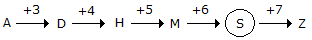Test: Letter Series Type 2 - Question 23

Directions to Solve

In each of the following questions, various terms of an alphabet series are given with one or more terms missing as shown by (?). Choose the missing terms out of the given alternatives.

Question -

PERPENDICULAR, ERPENDICULA, RPENDICUL, ?

Detailed Solution for Test: Letter Series Type 2 - Question 23

Each term of the series is obtained by removing two letters from the preceding term one from the beginning and one from the end, So, the missing term is PENDICU.

Test: Letter Series Type 2 - Question 24

Directions to Solve

In each of the following questions, various terms of an alphabet series are given with one or more terms missing as shown by (?). Choose the missing terms out of the given alternatives.

Question -

Z, ?, T, ?, N, ?, H, ?, B

Detailed Solution for Test: Letter Series Type 2 - Question 24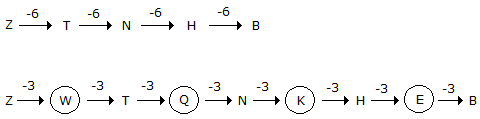Test: Letter Series Type 2 - Question 25

Directions to Solve

In each of the following questions, various terms of an alphabet series are given with one or more terms missing as shown by (?). Choose the missing terms out of the given alternatives.

Question -

ejo tyd ins xch ?

Detailed Solution for Test: Letter Series Type 2 - Question 25

There is a gap of four letters between the first and second, the second and third letters of each term, and also between the last letter of a term and the first letter of the next term.

Test: Letter Series Type 2 - Question 26

Directions to Solve

In each of the following questions, various terms of an alphabet series are given with one or more terms missing as shown by (?). Choose the missing terms out of the given alternatives.

Question -

Z, U, Q, ?, L

Detailed Solution for Test: Letter Series Type 2 - Question 26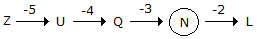Test: Letter Series Type 2 - Question 27

Directions to Solve

In each of the following questions, various terms of an alphabet series are given with one or more terms missing as shown by (?). Choose the missing terms out of the given alternatives.

Question -

M, N, O, L, R, I, V, ?

Detailed Solution for Test: Letter Series Type 2 - Question 27

The given sequence is a combination of two series:

I. M, O, R, V and II. N, L, I, ?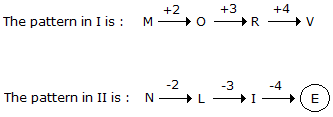Test: Letter Series Type 2 - Question 28

Directions to Solve

In each of the following questions, various terms of an alphabet series are given with one or more terms missing as shown by (?). Choose the missing terms out of the given alternatives.

Question -

Detailed Solution for Test: Letter Series Type 2 - Question 28

In the first step, one letter from the beginning and one from the end of a term are removed to give the next term. In the second step, two letters from the beginning of a term are removed.

These two steps are repeated alternately.

Test: Letter Series Type 2 - Question 29

Directions to Solve

In each of the following questions, various terms of an alphabet series are given with one or more terms missing as shown by (?). Choose the missing terms out of the given alternatives.

Question -

B, D, F, I, L, P, ?

Detailed Solution for Test: Letter Series Type 2 - Question 29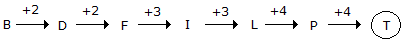Test: Letter Series Type 2 - Question 30

Directions to Solve

In each of the following questions, various terms of an alphabet series are given with one or more terms missing as shown by (?). Choose the missing terms out of the given alternatives.

Question -

DHL, PTX, BFJ, ?

Detailed Solution for Test: Letter Series Type 2 - Question 30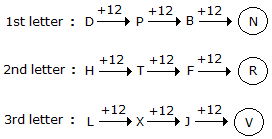## Logical Reasoning (LR) and Data Interpretation (DI)

96 videos|83 docs|119 tests
 Use Code STAYHOME200 and get INR 200 additional OFF Use Coupon Code
Information about Test: Letter Series Type 2 Page
In this test you can find the Exam questions for Test: Letter Series Type 2 solved & explained in the simplest way possible. Besides giving Questions and answers for Test: Letter Series Type 2, EduRev gives you an ample number of Online tests for practice

## Logical Reasoning (LR) and Data Interpretation (DI)

96 videos|83 docs|119 tests

### How to Prepare for UPSC

Read our guide to prepare for UPSC which is created by Toppers & the best Teachers